Get instant live expert help with Excel or Google Sheets“My Excelchat expert helped me in less than 20 minutes, saving me what would have been 5 hours of work!”

#### Post your problem and you’ll get expert help in seconds.

Your message must be at least 40 characters
Our professional experts are available now. Your privacy is guaranteed.

# Learn How to Calculate an IRR with a Terminal Value in Excel

Excel allows a user to calculate an IRR with a terminal value using the IRR function. This step by step tutorial will assist all levels of Excel users in getting an IRR of the free cash flow with the terminal value.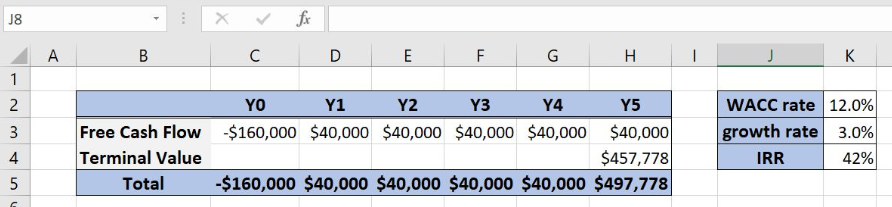Figure 1. The result of the IRR function

## Syntax of the IRR Formula

The generic formula for the IRR function is:

`=IRR (values, [guess])`

The parameters of the IRR function are:

• values – a range of cells containing values, including initial investment and incomes. The investment must have a negative sign, as it is a cost
• [guess] – an estimated value for the expected IRR. This parameter is non-mandatory. If it’s omitted, the function will take a default value of 0.1 (=10%).

## Setting up Our Data for the IRR Function with Terminal Value

Let’s look at the structure of the data we will use. In columns C:H, we have years 0-5. In rows, we have free cash flow without terminal value, while the terminal value will be calculated in the cell H4. Total cash flow with terminal value will be in the row “Total”. Finally, in the cell K4, we want to calculate the IRR for the specified cash-flow.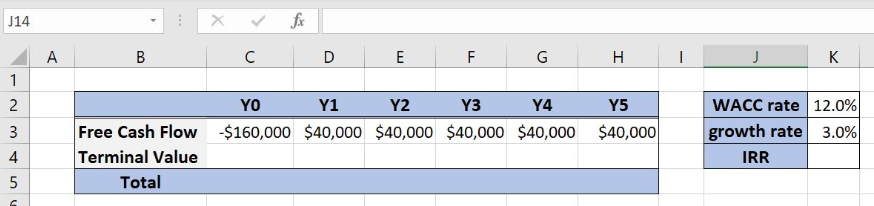Figure 2. Data that we will use in the example

## Get an IRR with a Terminal Value in Excel

In our example, we first want to calculate the terminal value based on the WACC rate, growth rate, and the cash flow in the Y5.

The formula looks like:

`=(H3*(1+K3))/(K2-K3)`

To apply the function, we need to follow these steps:

• Select cell H4 and click on it
• Insert the formula: `=(H3*(1+K3))/(K2-K3)`
• Press enter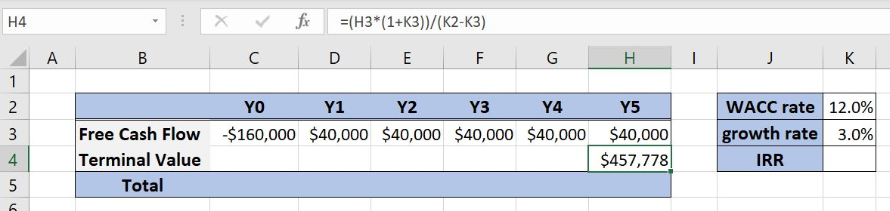Figure 3. Calculate a terminal value

Total Cash Flow is then calculated as a sum of the free cash flow and the terminal value:

The formula looks like:

`=C3+C4`

To apply the function, we need to follow these steps:

• Select cell C5 and click on it
• Insert the formula: `=C3+C4`
• Press enter
• Drag the formula right to the other cells in the row by clicking and dragging the little “+” icon at the bottom-right of the cell.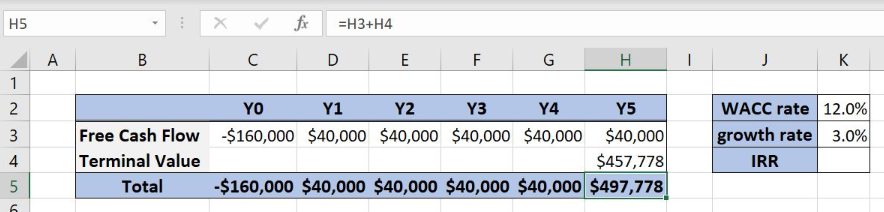Figure 4. Creating a free cash flow with a terminal value

Now, we can calculate the IRR using the IRR function and sums for every year.

The formula for IRR looks like:

`=IRR(C5:H5)`

The parameter values of the function is the range C5:H5.

To apply the IRR function, we need to follow these steps:

• Select cell K4 and click on it
• Insert the formula: `=IRR(C5:H5)`
• Press enter.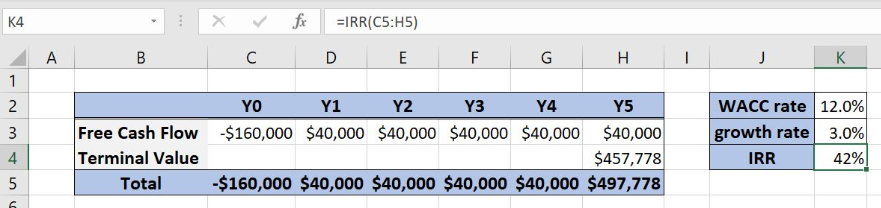Figure 5. Using the IRR function to calculate the IRR with a terminal value

Finally, the internal rate of return in the cell K4 is 42%.

Most of the time, the problem you will need to solve will be more complex than a simple application of a formula or function. If you want to save hours of research and frustration, try our live Excelchat service! Our Excel Experts are available 24/7 to answer any Excel question you may have. We guarantee a connection within 30 seconds and a customized solution within 20 minutes.